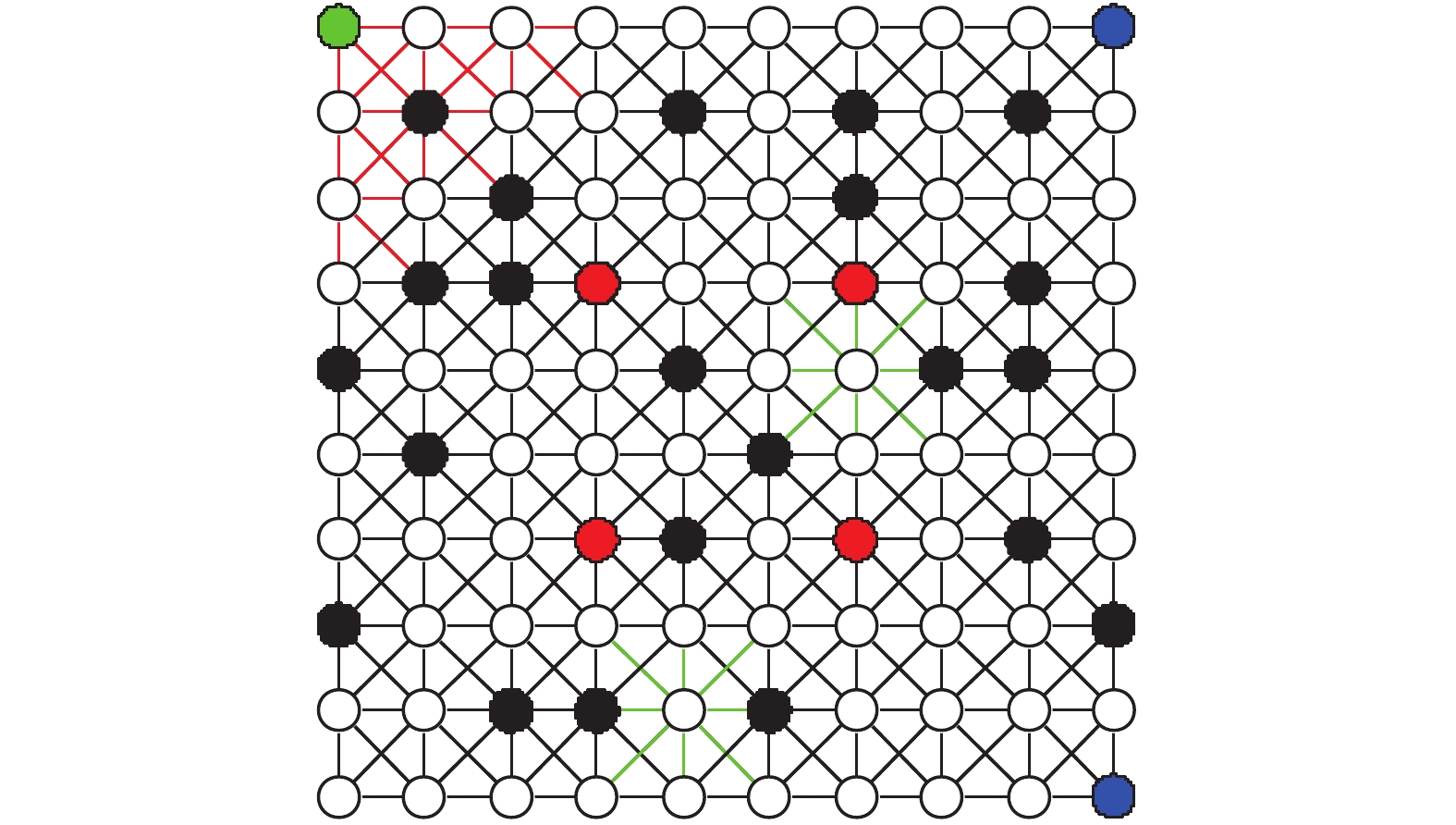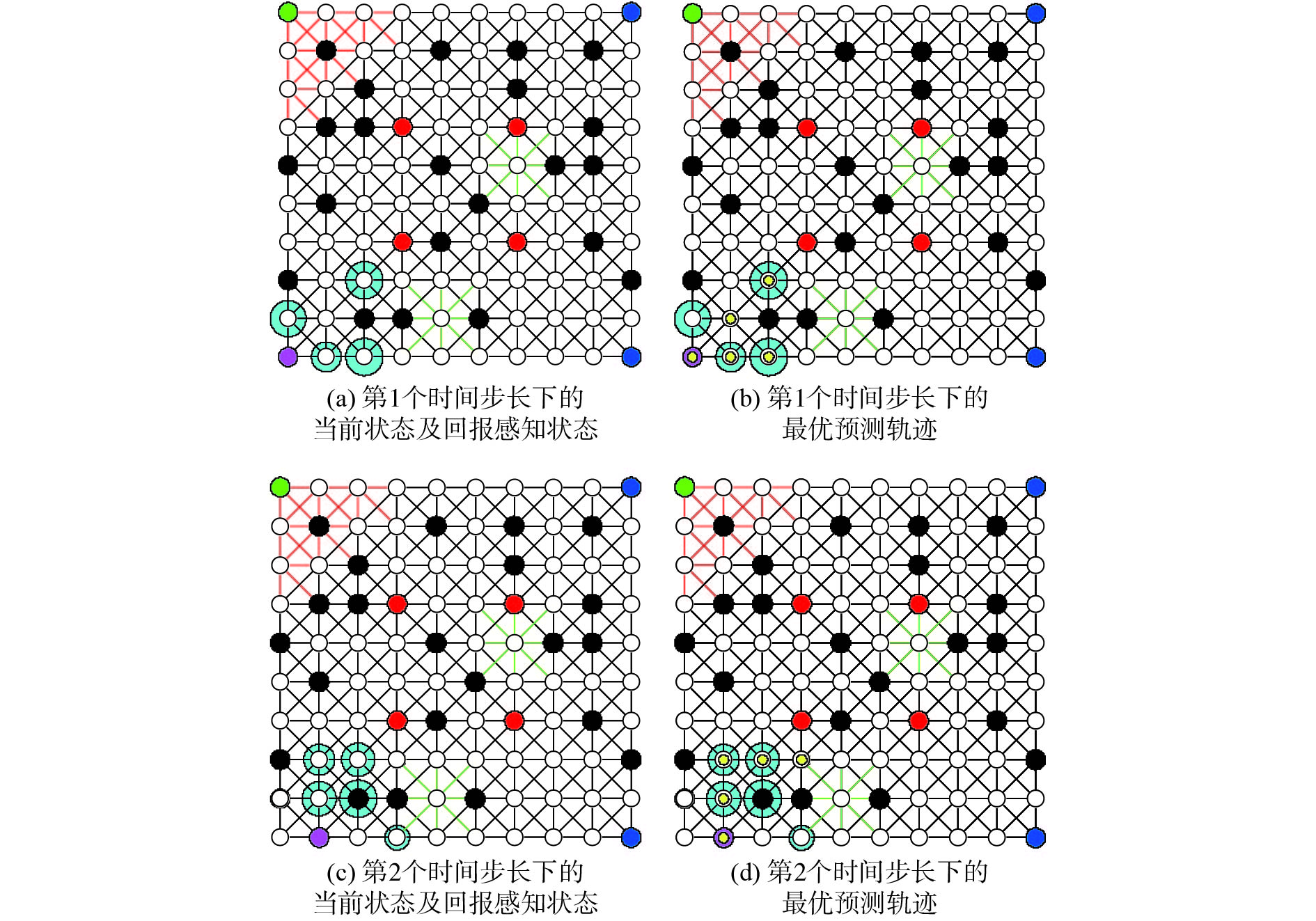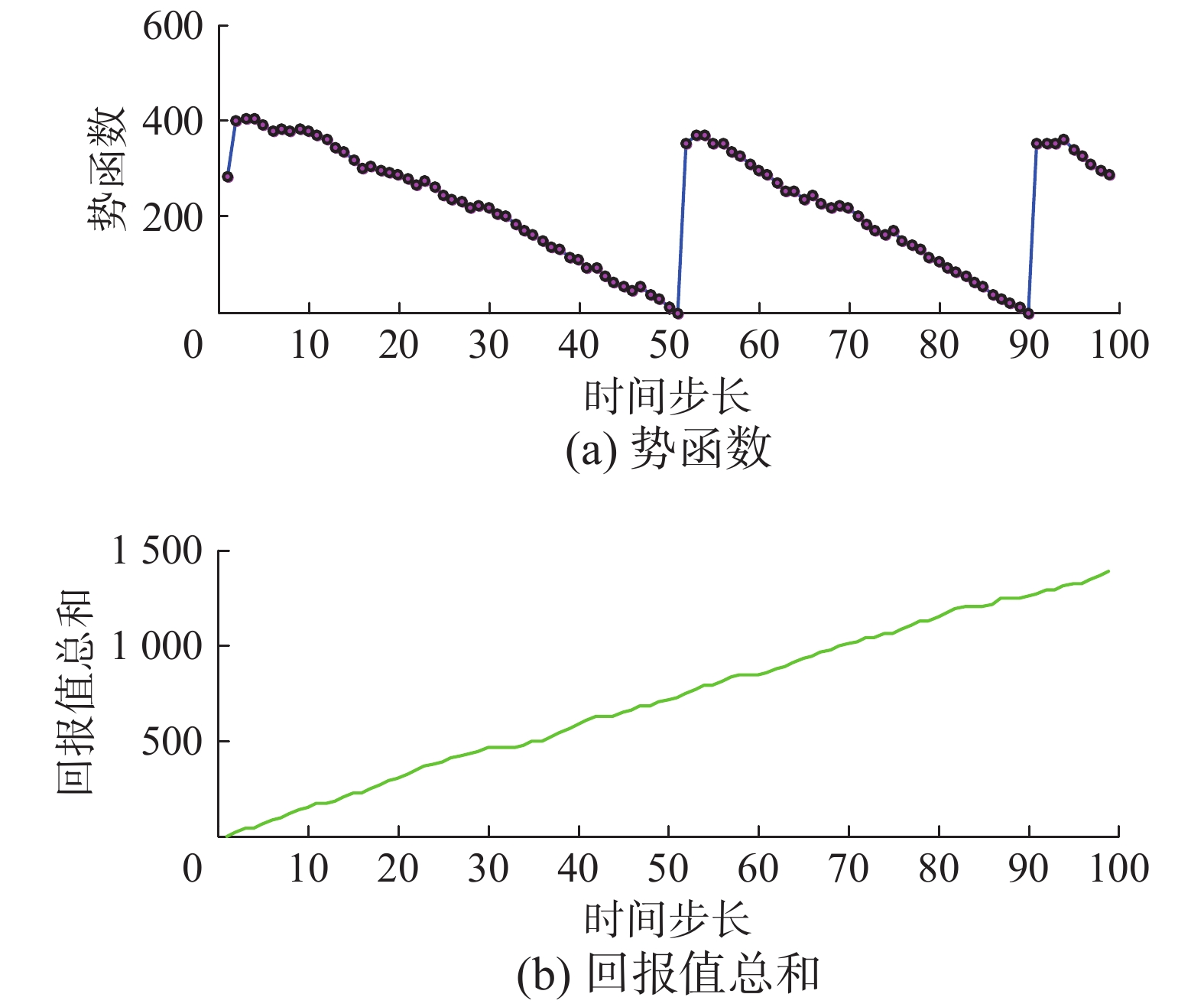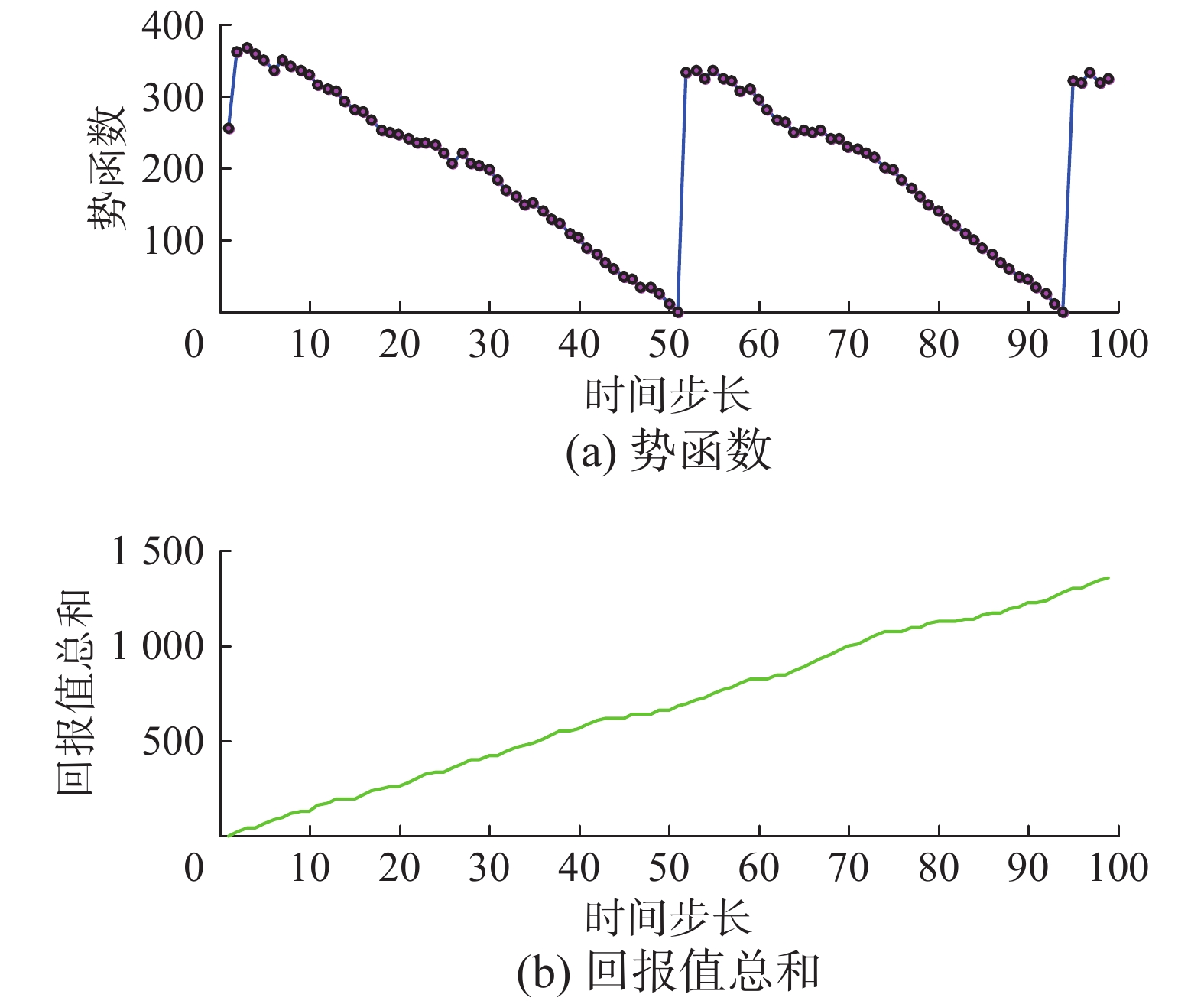﻿ 线性时序逻辑约束下的滚动时域控制路径规划
«上一篇文章快速检索 高级检索

 智能系统学报2020, Vol. 15Issue (2): 281-288  DOI: 10.11992/tis.2018100380

### 引用本文JIAO Mengtian, SONG Yunzhong. Receding horizon control path planning with linear temporal logic constraints[J]. CAAI Transactions on Intelligent Systems, 2020, 15(2): 281-288. DOI: 10.11992/tis.201810038.### 文章历史

Receding horizon control path planning with linear temporal logic constraints
JIAO Mengtian, SONG Yunzhong
School of Electrical Engineering and Automation, He’nan Polytechnic University, Jiaozuo 454003, China
Abstract: To address the path planning problem in finite deterministic systems, we propose a new method based on linear temporal logic constraints for the online real-time solution of receding horizon control. This method combines a receding horizon control method with a strategy that satisfies a linear temporal logic formula. The control objective is to maximize the collected reward while satisfying the high-level task specification, where the rewards are locally optimized at each time step over a finite horizon, and the immediate optimal control computed for the current time step is applied. By enforcing the appropriate constraints, the infinite trajectory produced by the controller is guaranteed to satisfy the desired temporal logic formula. Furthermore, by introducing the terrain factor, the results can be easily obtained. The simulation results indicate the feasibility and effectiveness of the proposed method.
Key words: linear temporal logic    receding horizon control    path planning    optimal control    finite deterministic systems    Büchi automaton    product automaton    terrain factor

1 预备理论

$Q$ 是状态的有限集合，每个状态代表道路网络中的一个节点；

${q_0} \in Q$ 是初始状态，代表初始位置；

$\Delta \subseteq Q \times Q$ 是切换函数，代表节点与节点之间切换关系的集合；

$\omega :\Delta \to {\rm{R}^ + }$ 是权重函数，为所有节点之间的有效切换分配一个正权值成本，如时间或距离等；

$\varPi$ 是观测状态的集合，即原子命题集合；

$h:Q \to {2^\varPi }$ 是观测图，即观测状态的命题函数。

${S\!\!_B}$ 是状态的有限集合；

${S\!\!_{B0}} \subseteq {S\!\!_B}$ 是初始状态的集合；

$\varSigma$ 是输入字母表；

$\delta :{S\!\!_B} \times \varSigma \to {2^{{S\!\!_B}}}$ 是切换函数；

${F\!\!_B} \subseteq S$ 是接受状态的集合。

 ${S\!\!_P} = Q \times {S\!\!_B}{\text{；}}$
 ${S\!\!_{P0}} = {\rm{\{ }}{q_0}{\rm{\} }} \times {S\!\!_{B0}}{\text{；}}$

${\Delta _P} \subseteq {S\!\!_P} \times {S\!\!_P}$ 是切换的集合，被定义为：如果 $q \to \varGamma q'$ $s\mathop \to \limits^{h(q)} Bs'$ $\left( {{\rm{(}}q,s{\rm{),(}}q',s'{\rm{)}}} \right) \in {\Delta _P}$

${\omega _P}{\rm{: }}{\Delta _P} \to {\rm{R}^ + }$ 是权重函数，被定义为

 ${\omega _P}((q,s),(q',s')) = \omega ((q,q')){\text{；}}$

${F_P} = Q \times {F_B}$ $P$ 上接受状态的集合。

 ${\gamma _\varGamma }({{p}}) = {{q}} = {q_0}{q_1}\cdots$ (1)
2 问题描述

2.1 切换系统Download: 图 1 给定的切换系统 Fig. 1 Given transition system

 $\rho {\rm{ = }}\left\{ \begin{gathered} 1.5,\;\;\;\;{\rm{10 < }}\omega (q,q') < 15 \\ 1.2,\;\;\;\;{\rm{ 0 < }}\omega (q,q') \leqslant 10{\rm{ }} \\ \end{gathered} \right.{\rm{ }}$ (2)

 $\rho {\rm{ = }}\left\{ \begin{gathered} 1.2,\;\;\;\;{\rm{10 < }}\omega (q,q') < 15 \\ 1,\;\;\;\;{\rm{0 < }}\omega (q,q') \leqslant 10{\rm{ }} \\ \end{gathered} \right.{\rm{ }}$ (3)

 $\rho {\rm{ = }}\left\{ \begin{gathered} 1.3,\;\;\;\;{\rm{10 < }}\omega (q,q') < 15 \\ 1.1,\;\;\;\;{\rm{0 < }}\omega (q,q') \leqslant 10{\rm{ }} \\ \end{gathered} \right.{\rm{ }}$ (4)

2.2 线性时序逻辑公式ϕ

 $\begin{gathered} {\rm{ }} \phi : ={\rm{G}}\neg a \\ \wedge {\rm{GFb}} \\ {\rm{ }} \wedge {\rm{G(b}} \to {\rm{X}}\neg {\rm{bUc)}} \\ {\rm{ }} \wedge {\rm{G(c}} \to {\rm{X}}\neg {\rm{cUd}}){\rm{ }} \\ \end{gathered}$ (5)

$\phi$ 的第一行， ${\rm{G}}\neg a$ 确保智能体在任何时间避免a观测状态。第二行， ${\rm{GFb}}$ 强制智能体重复访问观测状态b。第三行，确保智能体到达观测状态b后，并在返回状态b之前进入观测状态c。同样，第四行确保了智能体到达观测状态c之后并在重新回到c之前，智能体被驱动到观测状态d。即智能体要完成 ${{b}} \to {{c}} \to {{d}}$ 的监视任务。

2.3 假设的回报函数

1) 定义回报最大值为25

2) 定义回报最小值为10

3) 在 $k = 0$ 时刻：当 $\omega (q,q') \leqslant 29$ ，并且随机函数randi(2,1)≠1成立时，设置实际回报值在可取范围内随机产生

4) 在 $k > 0$ 时刻：当 $\omega (q,q') \leqslant 29$ 时，设置实际回报值在可取范围内随机产生

3 构建势函数

 $L({{p}}) = \sum\limits_{k = 1}^{n - 1} {\rho {\omega _P}} ({p_k},{p_{k + 1}})$ (6)

 $d(p,p') = \left\{ \begin{array}{l} \mathop {\min }\limits_{{{p}} \;\in D(p,\;p')} L({{p}}) \;{\text{若}}\;D({p_i},{p_j}) \ne {\text{Ø}} \\ \infty\;\;\;\;\;\;\;{\text{若}}\;D({p_i},{p_j}) = {\text{Ø}} \end{array} \right.$ (7)

 $V(p) = \left\{ \begin{array}{l} \mathop {\min }\limits_{p' \in F_P^*} {\rm{ }}d(p,p')\;\;{\text{若}}p \notin F_P^*\\ 0\;\;{\text{若}}p \in F_P^*{\rm{ }} \end{array} \right.$ (8)

1) 构建 ${\rm{B\ddot uchi}}$ 自动机： $B = {\rm LTL2BA}(\phi )$

2) 构建Product自动机： $P = T \otimes B$

3) 对于所有 $p \in {S_P}$ $p' \in {F_P}$ ，计算 $d(p,p')$

4) 设置 $F_P^ * = {F_P}$

5) 若存在 $q \in F_P^ *$ 使 $\mathop {\min }\limits_{p \;\in F_P^ * } d(q,p) = \infty$ ，则从 $F_P^ *$ 中移除 $q$ 以此获得最大自我可达集合 $F_P^ *$

6) 若 $F_P^ * {\rm{ = }}\text{Ø}$ ，则返回无满足任务的路径；

7) 对于所有 $p \in {S_P}$ ，使用定义(8)获得 ${{V}}(p)$

4 滚动时域控制器设计

 ${R_k}({{{p}}_k}) = \sum\limits_{i{\rm{ = }}1}^N {{R_k}({\gamma _\varGamma }({p_{i\;|k}}))}$ (9)
4.1 滚动时域控制器

4.1.1 k = 0时刻的滚动时域控制器

$k = 0$ 时刻，由于 $P$ 的初始状态不是唯一的，因此，可以从集合 ${S_{P0}} = \{ {q_0}\} \times {S_{B0}}$ 中选取任意初始初始状态。并将在初始时刻执行的控制器表示为 ${\operatorname{RH} ^0}({S_{P0}})$ ，定义如下:

 $\begin{array}{l} {{p}}_0^* = {{\mathop{\rm RH}\nolimits} ^0}({S_{P0}})\\ {\rm{ : = }}\mathop {{\rm{agrmax}}}\limits_{{p_0} \;\in {{P}}({p_0},N)} {R_0}({{{p}}_0}) \end{array}$ (10)

4.1.2 k > 0时刻的滚动时域控制器

$k > 0$ 时刻，控制器的形式表示为

 ${{p}}_k^* = \operatorname{RH} ({p_k},{{p}}_{k - 1}^{\rm{*}})$ (11)

 ${p_k} = p_{1|k - 1}^{\rm{*}},{\rm{ }}k = 1,2,\cdots$ (12)

 $\begin{array}{l} {{p}}_k^* = {\mathop{\rm RH}\nolimits} ({p_k},{{p}}_{k - 1}^{\rm{*}})\\ {\rm{ : = }}\mathop {{\bf{argmax}}}\limits_{{{{p}}_k} \in {{P}}({p_k},N)} {R_k}({{{p}}_k}) \end{array}$ (13)

 $\begin{array}{l} {{p}}_k^* = {\mathop{\rm RH}\nolimits} ({p_k},{{p}}_{k - 1}^{\rm{*}})\\ {\rm{ : = }}\mathop {{\bf{argmax}}}\limits_{{{{p}}_k} \in {{P}}({p_k},N)} {R_k}({{{p}}_k}) \end{array}$ (14)

 $\begin{array}{l} {{p}}_k^* = {\mathop{\rm RH}\nolimits} ({p_k},{{p}}_{k - 1}^{\rm{*}})\\ {\rm{ : = }}\mathop {{\bf{argmax}}}\limits_{{p_k} \in {{P}}({p_k},N)} {R_k}({{{p}}_k}){\rm{ }} \end{array}$ (15)

4.2 控制算法

1) 构建对应于 $\phi$ ${\rm{B\ddot uchi}}$ 自动机

 $B = {\rm{(}}{S_B},{S_{B0}},{2^\varPi }{\rm{,}}{\delta _B}{\rm{,}}{F_B}{\rm{)}};$

2) 构建Product自动机：

 $P = \varGamma \otimes B = {\rm{(}}{S_P},{S_{P0}},{\Delta _P},{\omega _P},{F_P}{\rm{)}};$

3) 计算所有状态 $p \in {S_p}$ 的势函数 ${{V}}(p)$

1) 若存在 ${p_0} \in {S_{P0}}$ 使 ${{V}}({p_0}) \ne \infty$ ，则设置 $k = 0$

2) 观测 $q \in \mathcal{N}({q_0},k)$ 邻域内各状态的回报值，获得 ${R_0}(q)$

3) 获得 ${{p}}_0^* = {\operatorname{RH} ^0}({S_{P0}})$

4) 在Product自动机 $P$ 上执行切换 $({p_0},p_{1|0}^*)$ ，在切换系统 $\varGamma$ 上执行 $({q_0},{\gamma _\varGamma }(p_{1|0}^ * ))$ 切换；

5) 设置 $k = 1$ ，进入循环；

6) 观测 $q \in \mathcal{N}({q_k},k)$ 邻域内各状态的回报值，获得 ${R_k}(q)$

7) 获得 ${{p}}_k^* = \operatorname{RH} ({p_k},{{p}}_{k - 1}^{\rm{*}})$

8) 在Product自动机 $P$ 上执行切换 $({p_k},p_{1|k}^*)$ ，在切换系统 $\varGamma$ 上执行 $({q_k},{\gamma _\varGamma }(p_{1|k}^ * ))$ 切换；

9) 设置 $k \leftarrow k + 1$

10) 结束循环；

11) 否则，将无源于 ${q_0}$ 的满足 $\phi$ 的运行路径；

12) 结束。

5 仿真结果分析Download: 图 2 在滚动时域控制律下系统轨迹的截图 Fig. 2 Screenshots of the system trajectory under the receding horizon control lawDownload: 图 3 100个时间步长下的势函数和回报值总和 Fig. 3 Potential function and total reward for 100 time-step

 $L({{p}}) = \sum\limits_{k = 1}^{n - 1} {{\omega _P}} ({p_k},{p_{k + 1}})$ (16)Download: 图 4 未引入地势影响因子时100个时间步长下的势函数和回报值总和 Fig. 4 Potential function and total reward for 100 time-step without terrain influence factor

6 结束语

  Fainekos G E, Girard A, Kress-Gazit H, et al. Temporal logic motion planning for dynamic robots[J]. Automatica, 2009, 45(2): 343-352. DOI:10.1016/j.automatica.2008.08.008 (0)  Plaku E, Karaman S. Motion planning with temporal logic specifications: progress and challenges[J]. AI communications, 2015, 29(1): 151-162. DOI:10.3233/AIC-150682 (0)  Saha S, Julius A A. Task and motion planning for manipulator arms with metric temporal logic specifications[J]. IEEE robotics and Automation letters, 2018, 3(1): 379-386. DOI:10.1109/LRA.2017.2755078 (0)  Andersen M S, Jensen R S, Bak T, et al. Motion planning in multi-robot systems using timed automata[J]. Promoting health for working women, 2004, 37(8): 597-602. (0)  Kloetzer M, Xuchu Ding, Belta C. Multi-robot deployment from LTL specifications with reduced communication[C]. The 50th IEEE conference on decision and control and European control conference. Orlando, USA, 2011: 4867–4872. (0)  Shital S. Chiddarwar, N. Ramesh Babu. Multi-agent system for off-line coordinated motion planning of multiple industrial robots[J]. International journal of advanced robotic systems, 2011, 8(1): 102-112. (0)  Karaman S, Frazzoli E. Vehicle routing with linear temporal logic specifications: applications to multi-UAV mission planning[J]. International journal of robust & Nonlinear control, 2011, 21(12): 1372-1395. (0)  Zhou Y, Maity D, Baras J S. Optimal mission planner with timed temporal logic constraints[C]. IEEE 2015 European control conference. Linz, Austria, 2015: 759-764. (0)  Chen Y, Ding X C, Stefanescu A, et al. Formal approach to the deployment of distributed robotic teams[J]. IEEE transactions on robotics, 2012, 28(1): 158-171. DOI:10.1109/TRO.2011.2163434 (0)  Ulusoy A, Smith S L, Ding X C, et al. Optimality and robustness in multi-robot path planning with temporal logic constraints[J]. International journal of robotics research, 2013, 32(8): 889-911. DOI:10.1177/0278364913487931 (0)  Guo M, Dimarogonas D V. Multi-agent plan reconfiguration under local LTL specifications[J]. International journal of robotics research, 2015, 34(2): 218-235. DOI:10.1177/0278364914546174 (0)  Dunbar W B, Caveney D S. Distributed receding horizon control of vehicle platoons: stability and string stability[J]. IEEE transactions on automatic control, 2012, 57(3): 620-633. DOI:10.1109/TAC.2011.2159651 (0)  李佩杰, 林颂晨, 白晓清, 等. 计及配电网三相模型的电动汽车充电滚动时域控制[J]. 中国电机工程学报, 2016, 36(17): 4533-4543. LI Peijie, LIN Songchen, BAI Xiaoqing, et al. Receding-horizon-control-based charging method of electric vehicle considering three-phase model of distribution network[J]. Proceedings of the CSEE, 2016, 36(17): 4533-4543. (0)  Grema A S, Cao Y. Receding horizon control for oil reservoir waterflooding process[J]. Systems science & Control engineering an open access journal, 2017, 5(1): 449-461. (0)  王文彬, 秦小林, 张力戈, 等. 基于滚动时域的无人机动态航迹规划[J]. 智能系统学报, 2018, 13(04): 524-533. WANG Wenbin, QIN Xiaolin, ZHANG Lige, et al. Dynamic UAV trajectory planning based on receding horizon[J]. CAAI transactions on intelligent systems, 2018, 13(04): 524-533. (0)  段海滨, 杨之元. 基于柯西变异鸽群优化的大型民用飞机滚动时域控制[J]. 中国科学: 技术科学, 2018, 48(03): 277-288. DUAN Haibin, YANG Zhiyuan. Large civil aircraft receding horizon control based on cauthy mutation pigeon inspired optimization[J]. Scientia Sinica technologica, 2018, 48(03): 277-288. (0)  Ulusoy A, Belta C. Receding horizon temporal logic control in dynamic environments[J]. The international journal of robotics research, 2014, 33(12): 1593-1607. DOI:10.1177/0278364914537008 (0)  Ding X C, Belta C, Cassandras C G. Receding horizon surveillance with temporal logic specifications[C]. The 49th IEEE conference on decision and control. Atlanta, USA, 2010: 256–261. (0)  Tumova J, Dimarogonas D V. A Receding horizon approach to multi-agent planning from local LTL specifications[C]. 2014 American control conference. Portland, USA, 2014: 1775–1780. (0)  Wongpiromsarn T, Topcu U, Murray R M. Receding horizon temporal logic planning for dynamical systems[C]. Proceedings of the 48th IEEE conference on decision and control. Shanghai, China, 2009, 57 (11): 5997–6004. (0)  Wongpiromsarn, Topcu, Ufuk, et al. Receding horizon control for temporal logic Specifications[C]. Proceedings of the 13th ACM international conference on hybrid systems. Stockholm, Sweden, 2010: 101–110. (0)  朱创营, 常亮, 徐周波, 等. 含有合取查询的时态描述逻辑ALC-LTL模型检测[J]. 智能系统学报, 2014, 9(06): 714-722. ZHU Chuangying, CHANG Liang, XU Zhoubo, et al. Research on the model checking of temporal description logic ALC-LTL containing conjunctive query[J]. CAAI transactions on intelligent systems, 2014, 9(06): 714-722. (0)  郭建, 边明明, 韩俊岗. LTL公式到自动机的转换[J]. 计算机科学, 2014, 9(06): 714-722. GUO Jian, BIAN Mingming, HAN Jungang. Translation from LTL formula into automata[J]. Computer science, 2014, 9(06): 714-722. (0)